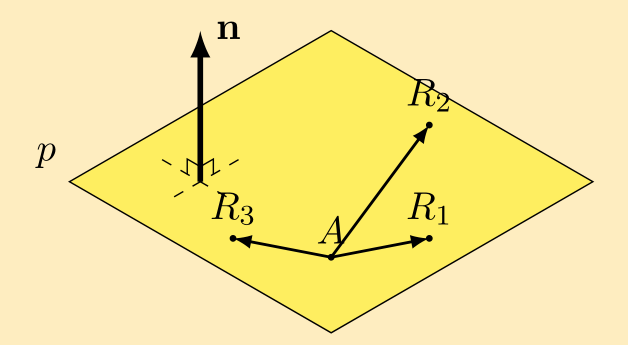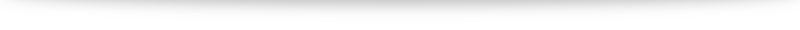# Why $\mathbf{r}\cdot\mathbf{n} = \mathbf{a}\cdot\mathbf{n}$ for planes?

When I was first learning about equations of planes, the vector form of a plane $\mathbf{r} = \mathbf{a} + \lambda \mathbf{d_1} + \mu \mathbf{d_2}$ made a lot more sense to me: pick different values of $\lambda$ and $\mu$, and we will end up at different points on the plane.

And while I can see why the vector form isn’t as useful for further applications because there are so many different direction vectors (infinite, in fact), and that the normal vector is a much better way to describe planes because it is unique (up to a scalar multiple), the equation $\mathbf{r}\cdot\mathbf{n} = \mathbf{a}\cdot\mathbf{n}$ just isn’t very intuitive and didn’t make a lot of sense. In today’s post I’d try my best to explain the origins of such an equation.

Understanding how the equation comes about boils down to knowing what $\mathbf{a}$ and $\mathbf{r}$ represent. $\mathbf{a}$ is the position vector of a fixed point $A$ that we already know lies on the plane. In forming an equation of an object, we will like to know what equation any other random points on the object satisfies. (For example, we say $y=2x+3$ is an equation of a line because we can check that $(0,3)$ satisfies the equation (and hence lies on the line) while $(1,4)$ does not). $\mathbf{r}$ is used to represent the position vector of a random point on our plane. We will call the point $R$.So, in the picture above, we have fixed $A$, and randomly pick a few points which we named $R_1, R_2$ and $R_3$. It turns out that, regardless of which $R$ we take, we can form a direction vector $\overrightarrow{AR}$. And the normal vector of our plane, $\mathbf{n}$ is so special in the sense that $\overrightarrow{AR}$ is perpendicular to $\mathbf{n}$. Thus we have $\overrightarrow{AR} \cdot \mathbf{n} = 0$.

The rest boils down to some algebra manipulation. $\overrightarrow{AR} = \overrightarrow{OR}-\overrightarrow{OA}$ so $(\mathbf{r}-\mathbf{a})\cdot \mathbf{n} = 0$. Expansion gives $\mathbf{r} \cdot \mathbf{n} – \mathbf{a} \cdot \mathbf{n} = 0$ which leads us to our familiar $\mathbf{r}\cdot\mathbf{n} = \mathbf{a}\cdot\mathbf{n}$.

Hopefully this proof and explanation helps, but I bet that even then the equation still looks a bit weird (it did to me years ago too!). The trick is to simply keep practicing with it and it’d become second nature in no time!